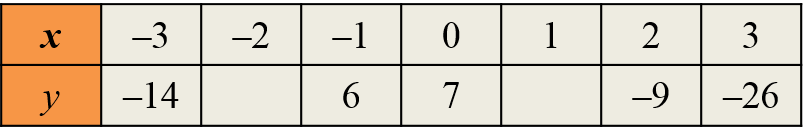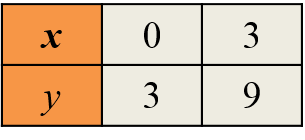# SPM Mathematics 2019, Paper 2 (Question 12)

Question 12:
(a) Complete Table 2 in the answer space, for the equation y = –3x2 – 2x + 7. [2 marks]

(b)
For this part of the question, use the graph paper. You may use a flexible curve rule.
Using a scale of 2 cm to 1 unit on the x-axis and 2 cm to 5 units on they-axis, draw the
graph of y = –3x2 – 2x + 7 for –3 < x < 3.       [4 marks]

(c) From graph 12(b), find
(i) the value of y when x = –0.5,
(ii) the value of x when y = –17.
[2 marks]

(d) Draw a suitable straight line on the graph in 12(b) to find the values of x which satisfy the equation 3x2 + 4x = 4 for –3 < x < 3. State the values of x.            [4 marks]Solution:
(a)
When x = –2,
y = –3(–2)2 – 2(–2) + 7
= –12 + 4 + 7
= –1

When x = 1,
y = –3(1)2 – 2(1) + 7
= –3 – 2 + 7
= 2

(b)(c)(i)
When x = –0.5; y = 7.0

(c)(ii)
When y = –17; x = 2.55

(d)
y = –3x2 – 2x + 7 …….. (1)
3x2 + 4x = 4
0 = –3x2 – 4x + 4 …….. (2)
(1) – (2) :  y = 2x + 3x = –2, x = 0.65